# SAT Math Multiple Choice Question 918: Answer and Explanation

### Test Information

Question: 918

2.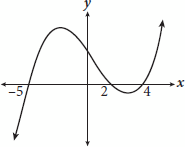The polynomial function shown in the graph crosses the x-axis at x = –5, x = 2, and x = 4. If the equation for this polynomial is written in the form y = ax3 + bx2 + cx + d, with a = 1, which of the following could be the equation?

• A.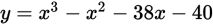• B.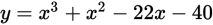• C.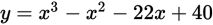• D.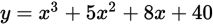Explanation:

C

Difficulty: Medium

Category: Passport to Advanced Math / Functions

Strategic Advice: You can use the x-intercepts shown in a graph to write the factors of the corresponding polynomial-here, there are three x-intercepts, so you should have three factors.

Getting to the Answer: You are given that a = 1, which tells you that each factor is of the form (x - a). To write the equation in the form requested, FOIL the first two factors, then multiply the result by the third factor. The order in which you multiply the factors doesn't matter. The function crosses the x-axis at x = -5, which means one factor is (x - (-5)), or (x + 5). The function also crosses the x-axis at x = 2 and x = 4, which translate to factors of (x - 2) and (x - 4). Start by FOILing (x + 5) and (x - 2). Then multiply the result by the remaining factor, (x - 4):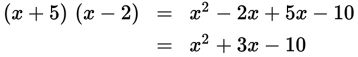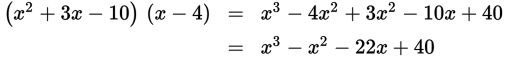This matches (C).